## Example Questions

### Example Question #1 : Number Line

How many integers are there between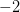and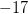(not includingor)?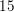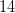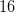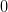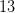Explanation:

Since we are not includingorin the count, the number of integers is given by the equation: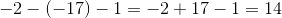If negative numbers are difficult for you to understand you can think of it this way. Since both values are negative and we are looking for the amount of integers between them we can solve the problem by subtracting 2 from 17 and then another one since we don't want to include the endpoints.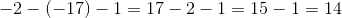### Example Question #1 : Integers

On a real number line, x1 = -4 and x2 = 14. What is the distance between these two points?

-18

10

18

4

18

Explanation:

The distance between two points is always positive. We calculate lx2 - x1l, which will give us the distance between the points.

|14- (-4)| = |14+4| = |18| = 18

### Example Question #1 : Number Lines And Absolute Value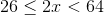Which of the following is a graph for the values ofdefined by the inequality stated above?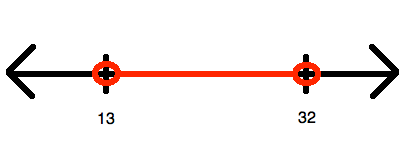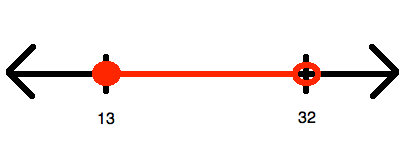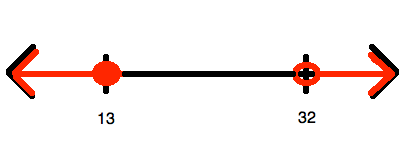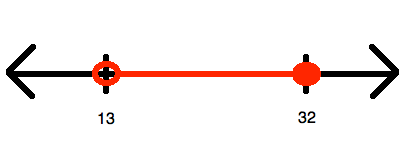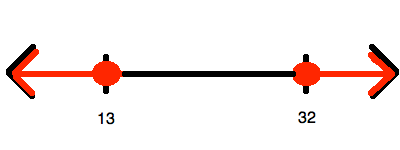Explanation:

To begin, you must simplify so that you "isolate", (i.e. at least eliminate any coefficients from it). To do this, divide all of the members of the inequality by: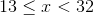Now, this inequality represents all of the numbers between 13 and 32.  However, it does include(hence, getting a closed circle for that value) and does not include(hence, getting an open circle for that value). Therefore, it looks like:### Example Question #1 : Graphing An Inequality With A Number Line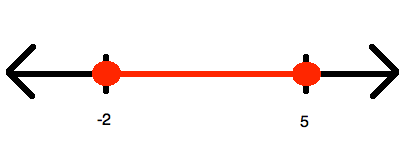Which of the following inequalities is represented by the number line shown above?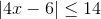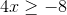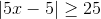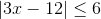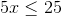Explanation:

Since the inequality represents one range of values between two end points (both of which are included, given the sign being "less than or equal"), you know that whatever you answer, it must be convertible to the form: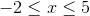Now, you know that it is impossible to get this out of the choices that have no absolute values involved in them. Therefore, the only options that make sense are the two having absolute values; however, here you should choose only the ones that have a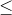, for only that will yield a range like this. Thus, we can try both of our options.

The wrong answer is simplified in this manner: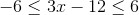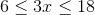And you can stop right here, for you know you will never havefor the left terminus.

The other option is simplified in this manner: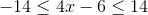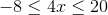This is just what you need!

### Example Question #1 : How To Graph An Inequality With A Number Line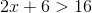Which of the following is a graph for the values ofdefined by the inequality stated above?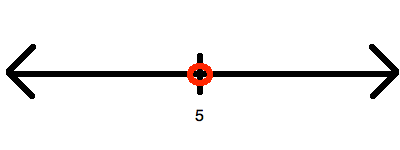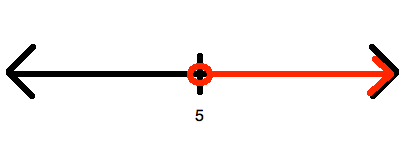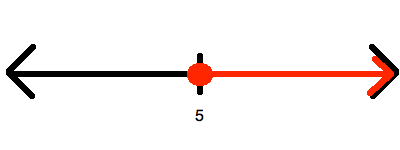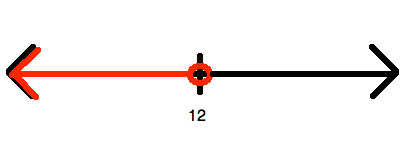Explanation:

Begin by solving for: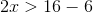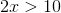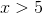Now, this is represented by drawing an open circle at 6 and graphing upward to infinity:### Example Question #1 : How To Find Value With A Number Line

On a real number line, what is the midpoint of –4 and 18?

4

8

14

7

11

7

Explanation:

The integer halfway between –4 and 18 is 7. The number 7 is 11 greater than –4 and 11 less than 18. Another way to determine this would be to find the average of the two numbers: (–4 + 18)/2 = 14/2 = 7.

### Example Question #1 : How To Find Value With A Number Line

On a number line, what is the distance between –6 and 7?

–1

–13

13

1

3

13

Explanation:

To find distance between two points, take the second and subtract the first.

So 7–(–6) = 7 + 6 = 13

### Example Question #1 : How To Find Value With A Number Line

What is the distance on a number line betweenand?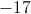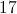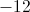Explanation:

The distance between 2 numbers on a number line is the sum of their absolute values.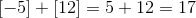### Example Question #1 : Number Line

Which equation can be used to describe the following number line: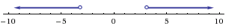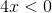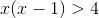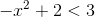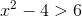Explanation:

Solve each equation for, plot on number line.

### Example Question #1 : How To Find Value With A Number Line

Which number below is farthest from –3 on a number line?

3

0

3/2

–8

–1